Question

# Suppose that Daniel has a 2.50 L bottle that contains a mixture of O2, N2, and...

Suppose that Daniel has a 2.50 L bottle that contains a mixture of O2, N2, and CO2 under a total pressure of 4.70 atm. He knows that the mixture contains 0.23 mol N2 and that the partial pressure of CO2 is 0.250 atm. If the temperature is 273 K, what is the partial pressure of O2?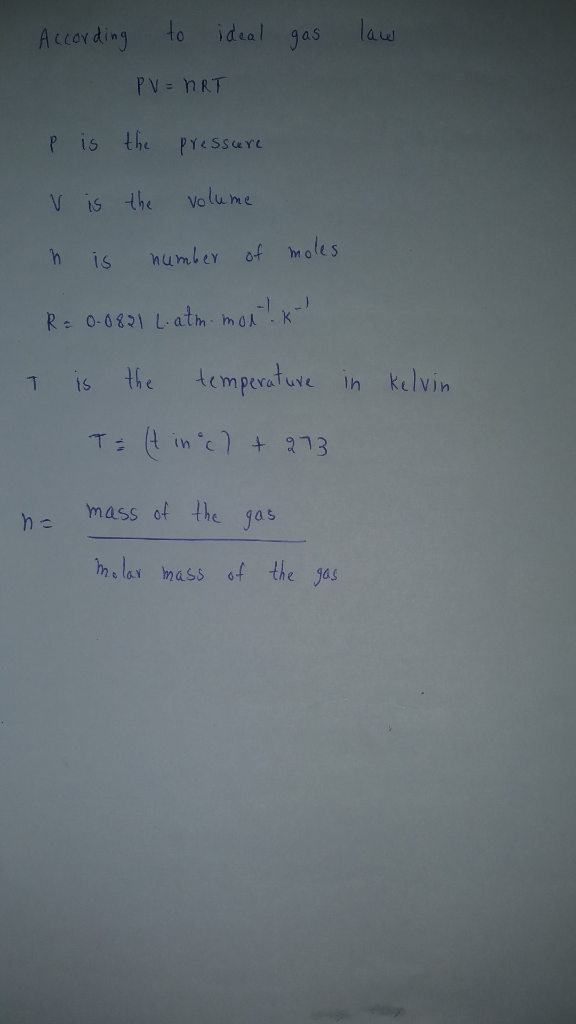Pressure due to nitrogen=(nN2*RT)/V

Pressure due to nitrogen=(0.23 mol*0.0821Latm mol-1 K-1*273K)/2.5L

Pressure due to nitrogen=2.062 atm

Pressure due to O2=total pressure-pressure of N2-pressure of CO2

Pressure due to O2=4.70-2.062-0.250 = 2.388 atm

#### Earn Coins

Coins can be redeemed for fabulous gifts.

Similar Homework Help Questions
• ### Suppose that Daniel has a 2.50 L bottle that contains a mixture of O2, N2, and...

Suppose that Daniel has a 2.50 L bottle that contains a mixture of O2, N2, and CO2 under a total pressure of 5.40 atm. He knows that the mixture contains 0.290 mol N2 and that the partial pressure of CO2 is 0.250 atm. If the temperature is 273 K, what is the partial pressure of O2? PO2= atm

• ### Suppose that Daniel has a 3.00 L bottle that contains a mixture of O2 , N2...

Suppose that Daniel has a 3.00 L bottle that contains a mixture of O2 , N2 , and CO2 under a total pressure of 5.30 atm. He knows that the mixture contains 0.310 mol N2 and that the partial pressure of CO2 is 0.350 atm. If the temperature is 273 K, what is the partial pressure of O2 ?

• ### Suppose that Daniel has a 2.50 L bottle that contains a mixture of O, N, and...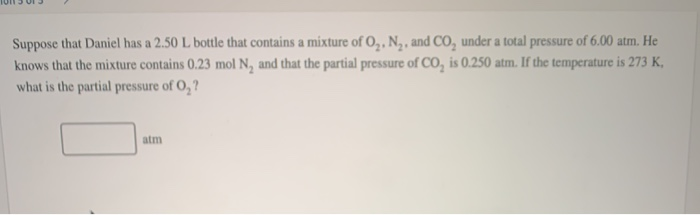Suppose that Daniel has a 2.50 L bottle that contains a mixture of O, N, and CO, under a total pressure of 6.00 atm. He knows that the mixture contains 0.23 mol N, and that the partial pressure of Co, is 0.250 atm. If the temperature is 273 K. what is the partial pressure of O,?

• ### Suppose that Daniel has a 2.50 L bottle that contains a mixture of 0,, N,, and...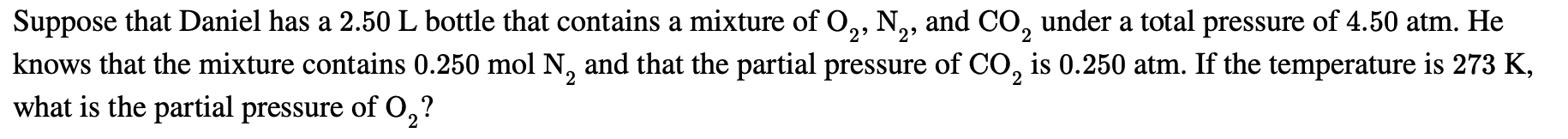Suppose that Daniel has a 2.50 L bottle that contains a mixture of 0,, N,, and CO, under a total pressure of 4.50 atm. He knows that the mixture contains 0.250 mol N, and that the partial pressure of CO, is 0.250 atm. If the temperature is 273 K, what is the partial pressure of 0,?

• ### Suppose that Daniel has a 2.50 L bottle that contains a mixture of O, N,, and...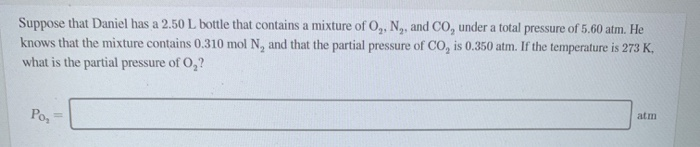Suppose that Daniel has a 2.50 L bottle that contains a mixture of O, N,, and CO, under a total pressure of 5.60 atm. He knows that the mixture contains 0.310 mol N, and that the partial pressure of Co, is 0.350 atm. If the temperature is 273 K. what is the partial pressure of O,? Pos

• ### Suppose that Daniel has a 2.50 L. bottle that contains a mixture of O.. N. and...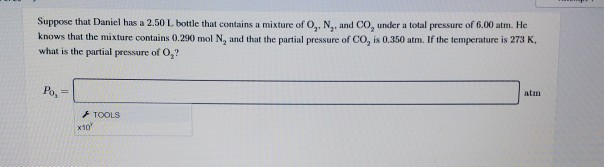Suppose that Daniel has a 2.50 L. bottle that contains a mixture of O.. N. and Counder a total pressure of 6.00 atm. He knows that the mixture contains 0.290 mol N, and that the partial pressure of Co, is 0.350 atm. If the temperature is 273 K, what is the partial pressure of O,? Po, - TOOLS

• ### Suppose that Daniel has a 3.00 L bottle that contains a mixture of O2O2, N2N2, and...

Suppose that Daniel has a 3.00 L bottle that contains a mixture of O2O2, N2N2, and CO2CO2 under a total pressure of 5.70 atm. He knows that the mixture contains 0.270 mol N2N2 and that the partial pressure of CO2CO2 is 0.250 atm. If the temperature is 273 K, what is the partial pressure of O2O2?

• ### Suppose that Daniel has a 2.00 L bottle that contains a mixture of O 2 ,...

Suppose that Daniel has a 2.00 L bottle that contains a mixture of O 2 , N 2 , and CO 2 under a total pressure of 5.50 atm. He knows that the mixture contains 0.250 mol N 2 and that the partial pressure of CO 2 is 0.350 atm. If the temperature is 273 K, what is the partial pressure of O 2 ?

• ### Suppose that Daniel has a 3.00 L bottle that contains a mixture of O, N,, and...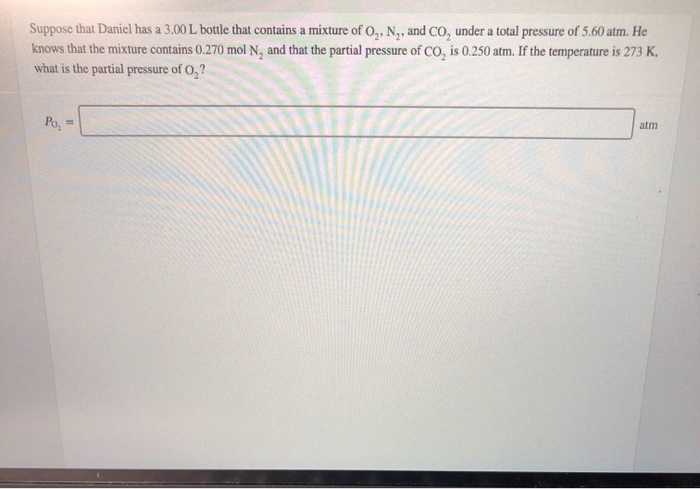Suppose that Daniel has a 3.00 L bottle that contains a mixture of O, N,, and Co, under a total pressure of 5.60 atm. He knows that the mixture contains 0.270 mol N, and that the partial pressure of Co, is 0.250 atm. If the temperature is 273 K, what is the partial pressure of 0,? Po, A sample of oxygen gas was collected via water displacement. Since the oxygen was collected via water displacement, the sample is saturated with...

• ### A student has a 2.68 L bottle that contains a mixture of O2 , N2 ,...

A student has a 2.68 L bottle that contains a mixture of O2 , N2 , and CO2 with a total pressure of 4.73 bar at 298 K . She knows that the mixture contains 0.273 mol N2 and that the partial pressure of CO2 is 0.306 bar . Calculate the partial pressure of O2 .